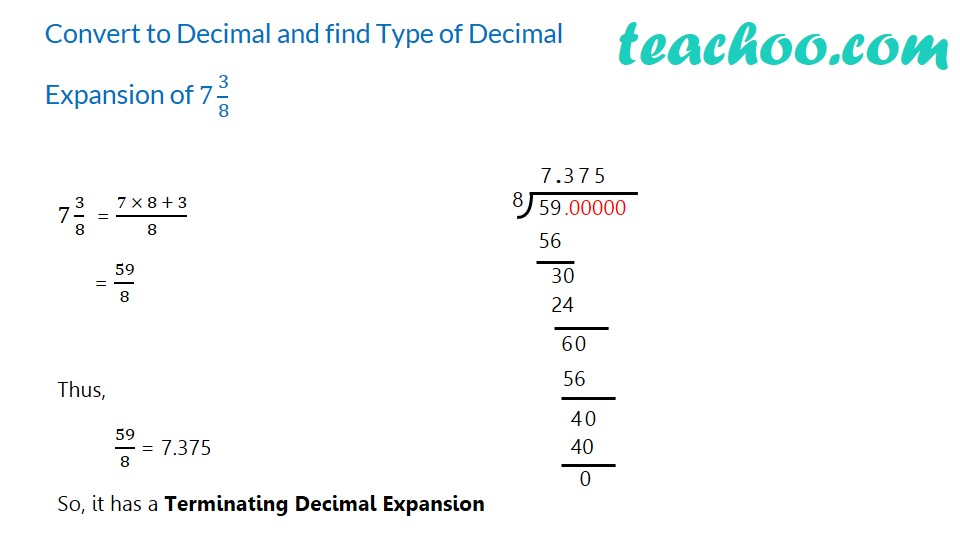1. Chapter 1 Class 9 Number Systems (Term 1)
2. Concept wise
3. Finding decimal expansion

Transcript

Convert to Decimal and find Type of Decimal Expansion of 7 3/8 7 3/8 = (7 × 8 + 3)/8 = 59/8 Thus, 59/8 = 7.375 So, it has a Terminating Decimal Expansion Try yourself…

Finding decimal expansion